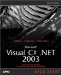Using the C Operators

Using the C# Operators

C# has the standard set of operators to manipulate your data, and you can see the C# operators arranged by category in Table 1.7. Operators work on operands and yield a value. For example, in the expression 5 + 3 , 5 is the first operand, + is the operator, 3 is the second operand, and this expression yields a value of 8. Just about all these operators are the same as in other languages, with some exceptions that we'll be seeing throughout the book.

As in C++, nearly all of the C# operators can be overloadedthat is, redefined for your own user -defined data types. More on this in Chapter 3.

FOR C++ PROGRAMMERS

Note that C# supports more operators than C++, such as is and typeof .

Table 1.7. The C# Operators by Category

CATEGORY

OPERATORS

Arithmetic

+ - * / %

Logical

& ^ ! ~ && true false

String concatenation

+

Increment, decrement

++ --

Shift

<< >>

Relational

== != < > <= >=

Assignment

= += -= *= /= %= &= = ^= <<= >>=

Member access

.

Indexing

[]

Cast

()

Conditional

?:

Delegate concatenation and removal

+ -

Object creation

new

Type information

is sizeof typeof

Overflow exception control

checked unch e cked

* -> [] &

Assignment Operators

We've already seen the most basic assignment operator at work, the = operator. This operator simply assigns a value to an lvalue (an lvalue is an element that corresponds to a location in memory that you can assign a value to, such as a variable):

class Assigner {   static void Main()   {  string text = "Hello from C#.";   System.Console.WriteLine(text);  } }

Besides the simple = assignment operator, C# also supports these compound assignment operators: += , -= , *= , /= , %= , &= , = , ^= , <<= , and >>= . As in C++ and Java, these operators combine an operation with an assignment. For example, the statement int1 += 5; adds 5 to the current value in int1 , and in logical terms is the same as the statement int1 = int1 + 5; .

Arithmetic Operators

The C# arithmetic operators are the same as you've seen elsewhere: + (addition), - (subtraction), * (multiplication), / (division), and % ( modulus , which returns the remainder after a divisionfor example, 16 % 3 equals 1). You use these operators as you would in other languages:

class Adder {   static void Main()   {  int value1 = 5, value2 = 10, result;   result = value1 + value2;  System.Console.WriteLine(result);   } }

Increment and Decrement Operators

As in C++ and Java, the increment and decrement operators, ++ and -- , take a little extra discussion, because they're different depending on whether you use them as prefix or postfix operators. You can use them as prefix operators as in ++variable , which increments the value in a variable before the rest of the statement is executed, or as postfix operators, as in variable++ , which increments the value in a variable after the rest of the statement has been executed. You can see an example showing both prefix and postfix usage in ch01_09.cs, Listing 1.9.

Listing 1.9 Prefix and Postfix Incrementing (ch01_09.cs)
class ch01_09 {   static void Main()   {     int value1 = 5, value2 = 5, result1, result2;     result1 = value1++;     result2 = ++value2;     System.Console.WriteLine(result1);     System.Console.WriteLine(result2);   } }

The code in ch01_09.cs gives you this result, where you can see that value1 wasn't incremented before its value was assigned to result1 , whereas value2 was incremented before the assignment:

C:\>ch01_09 5 6

We'll also take a look at the relational and logical operators when discussing the C# conditional statements later in this chapter.Microsoft Visual C#.NET 2003 Kick Start
ISBN: 0672325470
EAN: 2147483647
Year: 2002
Pages: 181

Similar book on Amazon